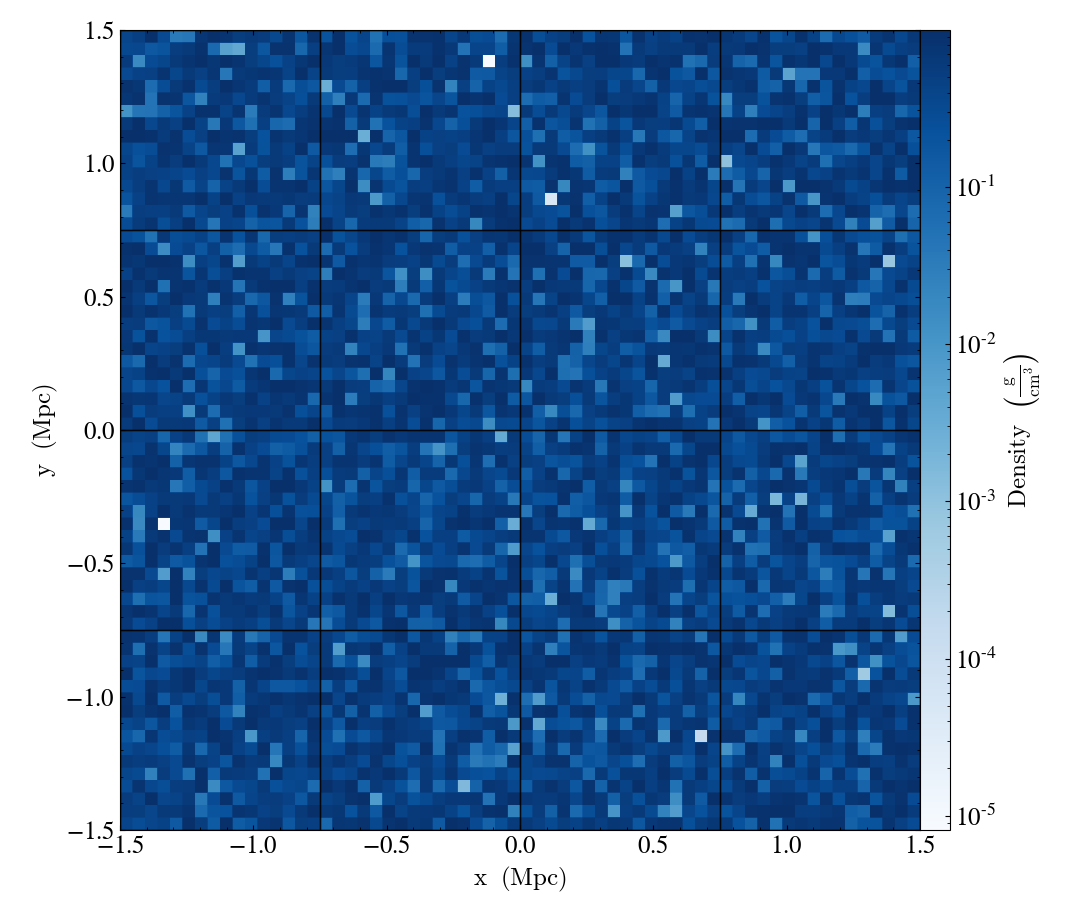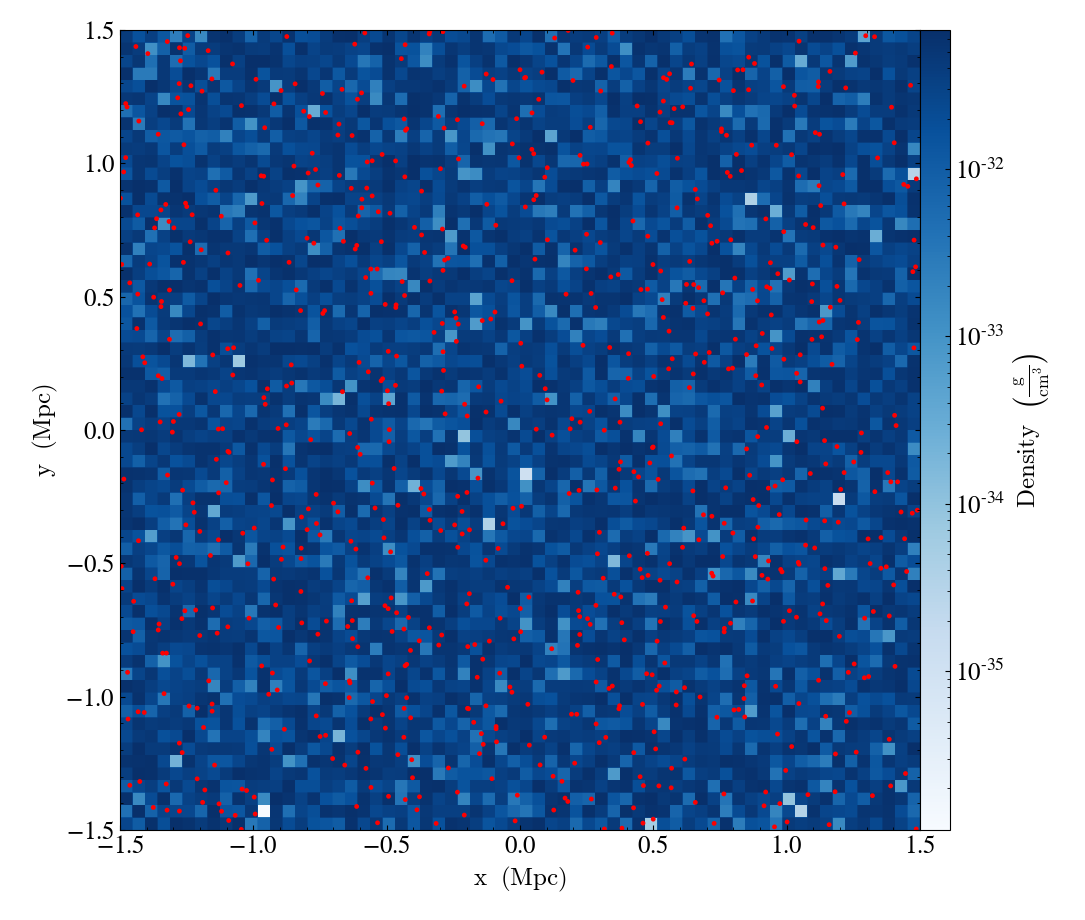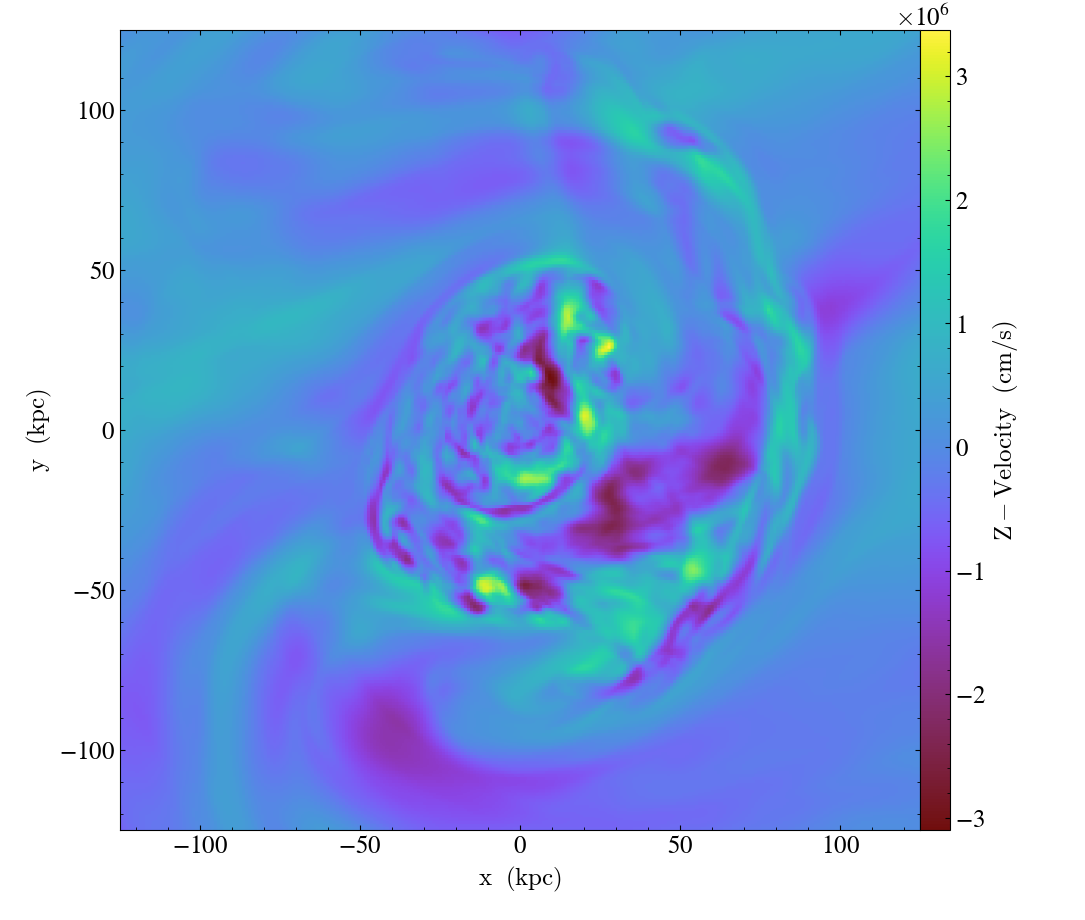Notebook

Even if your data is not strictly related to fields commonly used in astrophysical codes or your code is not supported yet, you can still feed it to yt to use its advanced visualization and analysis facilities. The only requirement is that your data can be represented as three-dimensional NumPy arrays with a consistent grid structure. What follows are some common examples of loading in generic array data that you may find useful.

## Generic Unigrid Data¶

The simplest case is that of a single grid of data spanning the domain, with one or more fields. The data could be generated from a variety of sources; we'll just give three common examples:

### Data generated "on-the-fly"¶

The most common example is that of data that is generated in memory from the currently running script or notebook.

In :
import numpy as np

import yt


In this example, we'll just create a 3-D array of random floating-point data using NumPy:

In :
arr = np.random.random(size=(64, 64, 64))


To load this data into yt, we need associate it with a field. The data dictionary consists of one or more fields, each consisting of a tuple of a NumPy array and a unit string. Then, we can call load_uniform_grid:

In :
data = dict(density=(arr, "g/cm**3"))
bbox = np.array([[-1.5, 1.5], [-1.5, 1.5], [-1.5, 1.5]])
ds = yt.load_uniform_grid(data, arr.shape, length_unit="Mpc", bbox=bbox, nprocs=64)


load_uniform_grid takes the following arguments and optional keywords:

• data : This is a dict of numpy arrays, where the keys are the field names
• domain_dimensions : The domain dimensions of the unigrid
• length_unit : The unit that corresponds to code_length, can be a string, tuple, or floating-point number
• bbox : Size of computational domain in units of code_length
• nprocs : If greater than 1, will create this number of subarrays out of data
• sim_time : The simulation time in seconds
• mass_unit : The unit that corresponds to code_mass, can be a string, tuple, or floating-point number
• time_unit : The unit that corresponds to code_time, can be a string, tuple, or floating-point number
• velocity_unit : The unit that corresponds to code_velocity
• magnetic_unit : The unit that corresponds to code_magnetic, i.e. the internal units used to represent magnetic field strengths. NOTE: if you want magnetic field units to be in the SI unit system, you must specify it here, e.g. magnetic_unit=(1.0, "T")
• periodicity : A tuple of booleans that determines whether the data will be treated as periodic along each axis

This example creates a yt-native dataset ds that will treat your array as a density field in cubic domain of 3 Mpc edge size and simultaneously divide the domain into nprocs = 64 chunks, so that you can take advantage of the underlying parallelism.

The optional unit keyword arguments allow for the default units of the dataset to be set. They can be:

• A string, e.g. length_unit="Mpc"
• A tuple, e.g. mass_unit=(1.0e14, "Msun")
• A floating-point value, e.g. time_unit=3.1557e13

In the latter case, the unit is assumed to be cgs.

The resulting ds functions exactly like a dataset like any other yt can handle--it can be sliced, and we can show the grid boundaries:

In :
slc = yt.SlicePlot(ds, "z", ("gas", "density"))
slc.set_cmap(("gas", "density"), "Blues")
slc.annotate_grids(cmap=None)
slc.show()Particle fields are detected as one-dimensional fields. Particle fields are then added as one-dimensional arrays in a similar manner as the three-dimensional grid fields:

In :
posx_arr = np.random.uniform(low=-1.5, high=1.5, size=10000)
posy_arr = np.random.uniform(low=-1.5, high=1.5, size=10000)
posz_arr = np.random.uniform(low=-1.5, high=1.5, size=10000)
data = dict(
density=(np.random.random(size=(64, 64, 64)), "Msun/kpc**3"),
particle_position_x=(posx_arr, "code_length"),
particle_position_y=(posy_arr, "code_length"),
particle_position_z=(posz_arr, "code_length"),
)
bbox = np.array([[-1.5, 1.5], [-1.5, 1.5], [-1.5, 1.5]])
data,
data["density"].shape,
length_unit=(1.0, "Mpc"),
mass_unit=(1.0, "Msun"),
bbox=bbox,
nprocs=4,
)


In this example only the particle position fields have been assigned. If no particle arrays are supplied, then the number of particles is assumed to be zero. Take a slice, and overlay particle positions:

In :
slc = yt.SlicePlot(ds, "z", ("gas", "density"))
slc.set_cmap(("gas", "density"), "Blues")
slc.annotate_particles(0.25, p_size=12.0, col="Red")
slc.show()### HDF5 data¶

HDF5 is a convenient format to store data. If you have unigrid data stored in an HDF5 file, it is possible to load it into memory and then use load_uniform_grid to get it into yt:

In :
from os.path import join

import h5py

from yt.config import ytcfg

data_dir = ytcfg.get("yt", "test_data_dir")
from yt.utilities.physical_ratios import cm_per_kpc

f = h5py.File(
join(data_dir, "UnigridData", "turb_vels.h5"), "r"


The HDF5 file handle's keys correspond to the datasets stored in the file:

In :
print(f.keys())

<KeysViewHDF5 ['Bx', 'By', 'Bz', 'Density', 'MagneticEnergy', 'Temperature', 'turb_x-velocity', 'turb_y-velocity', 'turb_z-velocity', 'x-velocity', 'y-velocity', 'z-velocity']>


We need to add some unit information. It may be stored in the file somewhere, or we may know it from another source. In this case, the units are simply cgs:

In :
units = [
"gauss",
"gauss",
"gauss",
"g/cm**3",
"erg/cm**3",
"K",
"cm/s",
"cm/s",
"cm/s",
"cm/s",
"cm/s",
"cm/s",
]


We can iterate over the items in the file handle and the units to get the data into a dictionary, which we will then load:

In :
data = {k: (v[()], u) for (k, v), u in zip(f.items(), units)}
bbox = np.array([[-0.5, 0.5], [-0.5, 0.5], [-0.5, 0.5]])

In :
ds = yt.load_uniform_grid(
data,
data["Density"].shape,
length_unit=250.0 * cm_per_kpc,
bbox=bbox,
nprocs=8,
periodicity=(False, False, False),
)


In this case, the data came from a simulation which was 250 kpc on a side. An example projection of two fields:

In :
prj = yt.ProjectionPlot(
ds, "z", ["z-velocity", "Temperature", "Bx"], weight_field="Density"
)
prj.set_log("z-velocity", False)
prj.set_log("Bx", False)
prj.show()